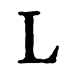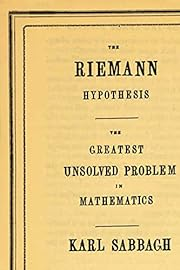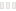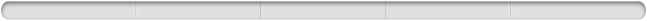Diese Seite verwendet Cookies für unsere Dienste, zur Verbesserung unserer Leistungen, für Analytik und (falls Sie nicht eingeloggt sind) für Werbung. Indem Sie LibraryThing nutzen, erklären Sie dass Sie unsere Nutzungsbedingungen und Datenschutzrichtlinie gelesen und verstanden haben. Die Nutzung unserer Webseite und Dienste unterliegt diesen Richtlinien und Geschäftsbedingungen.
Hide this

Auf ein Miniaturbild klicken, um zu Google Books zu gelangen.Lädt ...

# The Riemann Hypothesis: The Greatest Unsolved Problem in Mathematics

## vonKarl Sabbagh

 Mitglieder Rezensionen Beliebtheit Durchschnittliche Bewertung Diskussionen 207 4 103,113(4) 6 Since 1859, when the shy German mathematician Bernhard Riemann wrote an eight-page article giving a possible answer to a problem that had tormented mathematical minds for centuries, the world's greatest mathematicians have been fascinated, infuriated, and obsessed with proving the Riemann hypothesis. They speak of it in awed terms and consider it to be an even more difficult problem than Fermat's last theorem, which was finally proven by Andrew Wiles in 1995. InThe Riemann Hypothesis, acclaimed author Karl Sabbagh interviews some of the world's finest mathematicians who have spent their lives working on the problem--and whose approaches to meeting the challenges thrown up by the hypothesis are as diverse as their personalities. Wryly humorous, lively, accessible and comprehensive,The Riemann Hypothesis is a compelling exploration of the people who do math and the ideas that motivate them to the brink of obsession--and a profound meditation on the ultimate meaning of mathematics.… (mehr)
 Kürzlich hinzugefügt von sharedpresence, MarshaStanfield, kshultz, Rott, LesKoller, thoughtweaver, lidaskoteina, valcomm, QGCKeine
Lädt ...Melde dich bei LibraryThing an um herauszufinden, ob du dieses Buch mögen würdest.

Zeige 4 von 4
After having read Sabbagh’s book a General Solution to the Riemann Hypothesis popped up in my tiny little brain.

Here it is.

ζ(0) = negative one half = non trivial zero.

Proof:

1. Superimpose two unit circles.
2. Designate the vertical diameters as 2i and the horizontal diameters as 2x.
3. Starting at zero degrees and rotating counterclockwise, the points of axes along the circumference then assume the values (1, 0); (0, i); (-1, 0) and (0,-i).
4. Pin the common point of the two unit circles at (1; 0) such that this point is restricted from moving.
5. Reduce the diameter of one of the unit circles by half. This creates an inner circle of diameter i vertically and diameter x horizontally, which is pegged/pinned to the larger unit circle at point (1; 0):
6. This pegged inner circle is identical in every respect to the larger parent unit circle except it has been scaled to half the size of the larger unit circle.
7. The origin of the parent unit circle assumes various equivalent values Cartesian: zero, plus or minus 1

Fermatian: zero, limit, derivative, maxima, minima, plus and minus 1, one half, i
Newtonian: zero, shrinking the 2i secant to zero= i = limit = derivative = maxima = minima:
Inner Circle shift: The (-1; 0) point of the reduced circle now occupies the origin of the larger parent circle.

8. The origin of the reduced/inner circle is identical to the origin of the larger parent circle. They differ only in location.
9. Thus since i = 0 then the entirety of the i diameter of the reduced /inner circle is, and must be, zero.
10. The same result may be obtained by shrinking the 2i diameter/secant of the parent circle to the length of the i = zero diameter/secant of the inner circle.
11. Thus the i diameter comprises the critical line and since its entirety is composed of zeroes then these zeroes are, obligatorily, non-trivial zeroes.
12. Given step 7, i is equivalent to the tangent = m = slope.
13. Vis a vis the parent circle, this slope is manifest as the hypotenuse (tangent line) of a right triangle of height i and base .5x or one half x.
14. This results in the formation of a negative .5 slope or negative one half slope or a positive 2 precessional rate where slope = m = negative one half.
15. Therefore m = i = 0 = nontrivial zero = negative one half.
16. Therefore critical line equals
i = 0 = non trivial zero= negative one half = Zeta(0).
17. Thus is it proven that Zeta(0) =negative one half=non trivial zero.
QED

What people usually DON'T ask is the following: pick a random integer between 1 and N. What is the probability that the integer you picked is prime? In the polynomial case, they say: pick a random polynomial of degree d, with coefficients restricted. What is the probability that the polynomial I pick is irreducible?

It is known by the Prime Number Theorem that the fraction of integers between 1 and N that are prime is roughly 1 / log(N). Since the denominator grows toward infinity as N does, the probability that the integer is prime is very close to 0 when N is very large.

Some pointers not addressed in Sabbagh's book:

(1) Prime numbers are distributed throughout the infinite set of integers, where the number of primes below N is roughly ~ N/ln (N). The set of integers is well ordered by definition, i.e.: 1,2,3,4,5,6, . . . .
(2) IFF it were possible to similarly order the set (or some subset) of compact polynomials with integer coefficients, would the set of Prime polynomials in the larger set obey something like a logarithmic or exponential distribution? For instance: Where the of NON-prime polynomials might approach ln (N/N), and the density of Prime-polynomials might be ~ (N - ln (N/N)), or some powers of these?, i.e., the density of factorable polynomials decreases toward zero as N goes to infinity.

Has anyone ever regularized a polynomial version of the Riemann Zeta function for polynomials? I am not surprised no one hasn't since in some sense the algorithmic universe would have to be vast, or nature would be very simple. But I just don't know. I haven't been up to speed on Math Papers for the past years.

I'd have liked this exposition on the Riemann Hypothesis if Sabbagh had jnot concentrared so much on Louis de Branges efforts at proving it. Scary to think that so much was placed on the Riemann Hypothesis (all his life actually; this book was written in 2004; it's 2019 and no one has come nowhere close to solving it - forget Atiyah's attempt because it's bogus). It's probably impossible to understand the allure and the importance of the Riemann Hypothesis without some mathematical background, but if you have a decent first year college mathematical background, it's doable. Here's a more detailed view, also written by Sabbagh, on the de Branges attempt.

Bottom-line: A fun book. As another very concise but hugely influential example there's 'P=NP'. That's pretty easy to get on a t-shirt. And like the Riemann Hypothesis we're fairly sure what the outcome is ('P!=NP' in this case) but a proof could be very helpful. In the end, Math is too hard for mathematicians. They still cannot solve the Navier-Stokes equations. Engineers like myself routinely "solve" them by experimentation and clever approximations, the Reynolds-averaged Navier-Stokes equations that is. What do I care about proofs...? The Riemann Hypothesis really means that the primes are pretty much randomly distributed (with the probability of N being prime going like 1/log(N)), i.e., they're not concentrated and deconcentrated in certain waves. The proof of the Riemann Hypothesis could really be some clever analogy of the proof of the fact that there are infinitely many primes. If there were a finite set of primes, their products plus one would be another prime that would be a contradiction. A Riemann Hypothesis proof would be seismic. I do think, though, that the media would mainly ignore a valid proof. Very very few know who Riemann was outside of the math/physics community, let alone his hypothesis. If a proof of the Riemann Hypothesis is done through "traditional" ways I would expect it will be very hard to understand, and that one will also learn a lot of subtle complex analysis on the way. If the proof is easy because the approach is radically new, then I would expect that the approach turns out to be more interesting than the Riemann Hypothesis itself.

NB: ζ = Zeta Function. ()The funny thing about this book, for me, is that it claims to simply be a study of how the Riemann Hypothesis has been scrutinized, fought over and beloved by mathematicians. It is not supposed to be analysis of the theory itself. And yet for me, a non-mathematician, it has gotten me the closest to understanding the R.H.

And the anecdotes are simply great. ()
1Very gentle and readable intro to this million-dollar conjecture and the kind of people who would like to prove it.I have an undergraduate degree in mathematics from a long time ago, but haven't done a whole lot with it. Nevertheless, I gained something of an appreciation for the subject and am always interested when something important enough happens that it gets into the popular press. So naturally enough, I have been aware of the number one unsolved problem of mathematics, the Riemann hypothesis, and have followed the sporadic claims of its resolution over the last few decades.

Mr. Sabbagh's popular treatment of the problem in this book was a delight for me to read. He explains the hypothesis very clearly in a way that really doesn't even require any specialized knowledge of any arcane area of mathematics. Though great, this is not the primary virtue of the book. Rather it is his effort to reach out to the dozen or so mathematicians who are actively working on the problem who might have a hope of finally, after about a century and a half, of proving it. The reader is thus led to some appreciation of the world of the professional mathematician, with all of its human hopes and jealousies, striving to achieve a legacy that will outlive themselves. Sabbagh interviews them, some of them several times, attends their seminars, and listens for the inside dope that might show that someone somewhere is onto something.

The book is engaging, and I found it impossible to put down. It has lots of anecdotes, asides, and curiosities along the way to liven up the story. It is brutally honest in its portrayals of the principle characters. The writing style is lively, and the math is easy to follow. And it tells a story of man at his best--striving for progress, precision and truth. Quite the opposite of so many charlatans of the academy today, who seem to revel in ambiguity, imprecision, and political correctness. ()
2Zeige 4 von 4
Du musst dich einloggen, um "Wissenswertes" zu bearbeiten.
Weitere Hilfe gibt es auf der "Wissenswertes"-Hilfe-Seite.
Gebräuchlichster TitelOriginaltitelAlternative TitelUrsprüngliches ErscheinungsdatumFiguren/CharaktereWichtige SchauplätzeWichtige EreignisseZugehörige FilmePreise und AuszeichnungenEpigraph (Motto/Zitat)WidmungErste WorteZitateLetzte WorteHinweis zur IdentitätsklärungVerlagslektorenWerbezitate vonOriginalspracheAnerkannter DDC/MDSAnerkannter LCCLiteraturhinweise zu diesem Werk aus externen Quellen.

##Wikipedia auf Englisch (1)

 Riemann hypothesis
Since 1859, when the shy German mathematician Bernhard Riemann wrote an eight-page article giving a possible answer to a problem that had tormented mathematical minds for centuries, the world's greatest mathematicians have been fascinated, infuriated, and obsessed with proving the Riemann hypothesis. They speak of it in awed terms and consider it to be an even more difficult problem than Fermat's last theorem, which was finally proven by Andrew Wiles in 1995. InThe Riemann Hypothesis, acclaimed author Karl Sabbagh interviews some of the world's finest mathematicians who have spent their lives working on the problem--and whose approaches to meeting the challenges thrown up by the hypothesis are as diverse as their personalities. Wryly humorous, lively, accessible and comprehensive,The Riemann Hypothesis is a compelling exploration of the people who do math and the ideas that motivate them to the brink of obsession--and a profound meditation on the ultimate meaning of mathematics.

Keine Bibliotheksbeschreibungen gefunden.

BuchbeschreibungZusammenfassung in Haiku-Form## Melvil Decimal System (DDC)

512.73 — Natural sciences and mathematics Mathematics Algebra Number theory

## Bewertung

Durchschnitt:(4)
 0.5 1 1.5 2 2.5 31 3.5 49 4.5 51

## Bist das du?

Werde ein LibraryThing-Autor.

Über uns | Kontakt/Impressum | LibraryThing.com | Datenschutz/Nutzungsbedingungen | Hilfe/FAQs | Blog | LT-Shop | APIs | TinyCat | Nachlassbibliotheken | Vorab-Rezensenten | Wissenswertes | 163,344,805 Bücher! | Menüleiste: Immer sichtbar